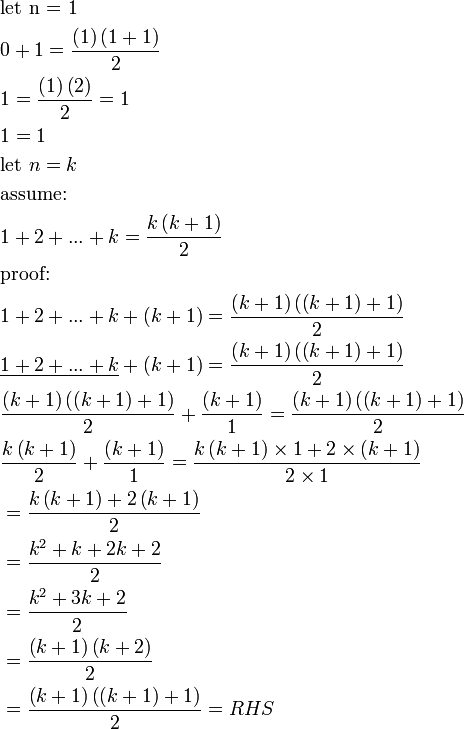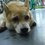# Mathematical Induction PART 2In Part 1

It shows that

Step 1 is Show it is true for n=1

Step 2 is Show that if n=k is true then n=k+1 is also true

so How to Do it?

Step 1 : * prove* it is true for n=1 (ｎｏｒｍａｌｌｙ）

Step 2 　：　 done　in this way normally we can prove it out..

First ~ Assume it is true forn=k

Second ~ Prove it is true for n=k+1 (normally we can use the n=k case as fact )

S (n / k) must be all positive integers including n/k

EXAMPLE

PROVE 1 + 3 + 5 + ... + (2n-1) = n^2

Fisrt : Show it is true for n=1

from LEFT : 1+3+5+.....+(2(1)-1) = 1

from RIGHT n^2 = (1^2) =1 SO 1 = 1^2 is True

SECOND : Assume it is true for n=k

1 + 3 + 5 + ... + (2^k-1) = k^2 is True

(prove it by yourself :) write down your steps in comment )

THIRD : prove it is true for k+1

1 + 3 + 5 + ... + (2^k-1) + (2^(k+1)-1) = (k+1)^2 ... ? (prove it by yourself :) write down your steps in comment )

We know that 1 + 3 + 5 + ... + (2k-1) = k^2 (the assumption above), so we can do a replacement for all but the last term:

k^2 + (2(k+1)-1) = (k+1)^2

THEN expanding all terms:

LEFT : k^2 + 2k + 2 - 1 = k^2 + 2k+1

NEXT simplifying :

RIGHT :k^2 + 2k + 1 = k^2 + 2k + 1

LEFT and RIGHT are the same! So it is true, it is proven.

THEREFORE :

1 + 3 + 5 + ... + (2(k+1)-1) = (k+1)^2 is TRUE!!!!!!

Mathematical Induction IS done !!! :)Note by Nicole Ling
6 years, 6 months ago

This discussion board is a place to discuss our Daily Challenges and the math and science related to those challenges. Explanations are more than just a solution — they should explain the steps and thinking strategies that you used to obtain the solution. Comments should further the discussion of math and science.

When posting on Brilliant:

• Use the emojis to react to an explanation, whether you're congratulating a job well done , or just really confused .
• Ask specific questions about the challenge or the steps in somebody's explanation. Well-posed questions can add a lot to the discussion, but posting "I don't understand!" doesn't help anyone.
• Try to contribute something new to the discussion, whether it is an extension, generalization or other idea related to the challenge.

MarkdownAppears as
*italics* or _italics_ italics
**bold** or __bold__ bold
- bulleted- list
• bulleted
• list
1. numbered2. list
1. numbered
2. list
Note: you must add a full line of space before and after lists for them to show up correctly
paragraph 1paragraph 2

paragraph 1

paragraph 2

[example link](https://brilliant.org)example link
> This is a quote
This is a quote
    # I indented these lines
# 4 spaces, and now they show
# up as a code block.

print "hello world"
# I indented these lines
# 4 spaces, and now they show
# up as a code block.

print "hello world"
MathAppears as
Remember to wrap math in $$ ... $$ or $ ... $ to ensure proper formatting.
2 \times 3 $2 \times 3$
2^{34} $2^{34}$
a_{i-1} $a_{i-1}$
\frac{2}{3} $\frac{2}{3}$
\sqrt{2} $\sqrt{2}$
\sum_{i=1}^3 $\sum_{i=1}^3$
\sin \theta $\sin \theta$
\boxed{123} $\boxed{123}$

Sort by:

calculus?

- 6 years, 3 months ago

so it is math

- 6 years, 3 months ago

really?

- 6 years, 3 months ago

what

- 6 years, 3 months ago

roar

- 6 years, 3 months ago

feeling lucky

- 6 years, 3 months ago

feeling happy

- 6 years, 3 months ago

- 6 years, 3 months ago

tiger

- 6 years, 3 months ago

is that true?

- 6 years, 3 months ago

yes

- 6 years, 3 months ago

no

- 6 years, 3 months ago

What have you been telling?

- 6 years, 3 months ago

what is your question ? I can't understand what did you want to say through your comments..

- 6 years, 2 months ago

a

- 6 years, 3 months ago

good

- 6 years, 3 months ago

nice

- 6 years, 3 months ago